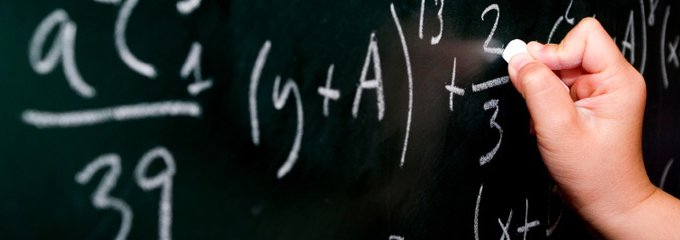Student Support

# Math Workshops## Math Workshop Information  - Fall 2020 - INFO COMING SOON

SMC will host FREE Math Success Intensives to help our students succeed in Math ## classes. These workshops will allow students to work in small groups with a faculty member and student tutors to gain the math skills that you will need to excel in your next math class.

Save time and money by allowing SMC math faculty and student math tutors to prepare you for success!

## Math Workshop Schedule - Fall 2020 -

Week Date Math 7 (MC 73) Math 50 (MC 73)
3   Limits, and Continuity Ratios, Percent, Exponents, Order of Operations, and Scientific Notation
4   Derivatives, and Rates of Change Solving Linear Equations in One Variable
5   The Derivative as a Function, and Differentiation Formulas Solving (Stats) Formulas and Solving Problems (Proportion & Percent)
6   The Chain Rule, and Implicit Differentiation Intro to Stats (definitions, sampling methods, and type of studies)
7   Related Rates Frequency Tables, Bar Graphs, Pie Charts, Two-Way Tables, Histograms
8   Maximum and Minimum Values, and The Mean Value Theorem Measures of Center, and Measures of Spread
9   Summary of Curve Sketching, and Optimization Problems Boxplots, and Graphing Equations of Lines
10   Antiderivatives, and Areas and Distances Rate of Change and Slope of a Line, and Find an Equation of a Line
11   The Definite Integral Scatterplots, Associations, and Modeling Linear Associations
12   The Fundamental Theorem of Calculus Integer and Rational Exponents, and Exponential Functions
13   The Substitution Rule, and Area Between Curves Logarithmic Functions, and System of Equations
14   Thanksgiving Holiday - CAMPUS CLOSED
15   Volumes, Work, and Average Values of a Function Solving Systems of Equations and Probability